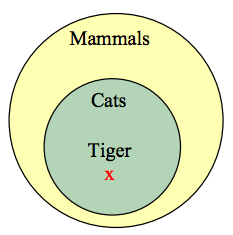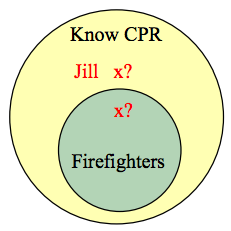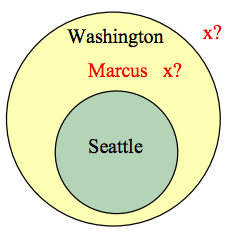## Arguments

### Learning Outcomes

• Discern between an inductive argument and a deductive argument
• Evaluate deductive arguments
• Analyze arguments with Venn diagrams and truth tables
• Use logical inference to infer whether a statement is true
• Identify logical fallacies in common language including appeal to ignorance, appeal to authority, appeal to consequence, false dilemma, circular reasoning, post hoc, correlation implies causation, and straw man arguments

A logical argument is a claim that a set of premises support a conclusion. There are two general types of arguments: inductive and deductive arguments.

### Argument types

An inductive argument uses a collection of specific examples as its premises and uses them to propose a general conclusion.

A deductive argument uses a collection of general statements as its premises and uses them to propose a specific situation as the conclusion.

### Example

The argument “when I went to the store last week I forgot my purse, and when I went today I forgot my purse. I always forget my purse when I go the store” is an inductive argument.

The premises are:

I forgot my purse last week
I forgot my purse today

The conclusion is:

I always forget my purse

Notice that the premises are specific situations, while the conclusion is a general statement. In this case, this is a fairly weak argument, since it is based on only two instances.

### Example

The argument “every day for the past year, a plane flies over my house at 2pm. A plane will fly over my house every day at 2pm” is a stronger inductive argument, since it is based on a larger set of evidence.

### Evaluating inductive arguments

An inductive argument is never able to prove the conclusion true, but it can provide either weak or strong evidence to suggest it may be true.

Many scientific theories, such as the big bang theory, can never be proven. Instead, they are inductive arguments supported by a wide variety of evidence. Usually in science, an idea is considered a hypothesis until it has been well tested, at which point it graduates to being considered a theory. The commonly known scientific theories, like Newton’s theory of gravity, have all stood up to years of testing and evidence, though sometimes they need to be adjusted based on new evidence. For gravity, this happened when Einstein proposed the theory of general relativity.

A deductive argument is more clearly valid or not, which makes them easier to evaluate.

### Evaluating deductive arguments

A deductive argument is considered valid if all the premises are true, and the conclusion follows logically from those premises. In other words, the premises are true, and the conclusion follows necessarily from those premises.

### Example

The argument “All cats are mammals and a tiger is a cat, so a tiger is a mammal” is a valid deductive argument.

The premises are:

All cats are mammals
A tiger is a cat

The conclusion is:

A tiger is a mammal

Both the premises are true. To see that the premises must logically lead to the conclusion, one approach would be use a Venn diagram. From the first premise, we can conclude that the set of cats is a subset of the set of mammals. From the second premise, we are told that a tiger lies within the set of cats. From that, we can see in the Venn diagram that the tiger also lies inside the set of mammals, so the conclusion is valid.### Analyzing Arguments with Venn/Euler diagrams

To analyze an argument with a Venn/ Euler diagram

1. Draw a Venn/ Euler diagram based on the premises of the argument
2. If the premises are insufficient to determine what determine the location of an element, indicate that.
3. The argument is valid if it is clear that the conclusion must be true

### Example

 Premise: All firefighters know CPR Premise: Jill knows CPR Conclusion: Jill is a firefighter

From the first premise, we know that firefighters all lie inside the set of those who know CPR. From the second premise, we know that Jill is a member of that larger set, but we do not have enough information to know if she also is a member of the smaller subset that is firefighters.Since the conclusion does not necessarily follow from the premises, this is an invalid argument, regardless of whether Jill actually is a firefighter.

It is important to note that whether or not Jill is actually a firefighter is not important in evaluating the validity of the argument; we are only concerned with whether the premises are enough to prove the conclusion.

In addition to these categorical style premises of the form “all ___,” “some ____,” and “no ____,” it is also common to see premises that are implications.

### Example

 Premise: If you live in Seattle, you live in Washington. Premise: Marcus does not live in Seattle. Conclusion: Marcus does not live in Washington.

From the first premise, we know that the set of people who live in Seattle is inside the set of those who live in Washington. From the second premise, we know that Marcus does not lie in the Seattle set, but we have insufficient information to know whether or not Marcus lives in Washington or not. This is an invalid argument.### Example

Consider the argument “You are a married man, so you must have a wife.”

Some arguments are better analyzed using truth tables.

### Example

Consider the argument:

 Premise: If you bought bread, then you went to the store Premise: You bought bread Conclusion: You went to the store

### Analyzing arguments using truth tables

To analyze an argument with a truth table:

1. Represent each of the premises symbolically
2. Create a conditional statement, joining all the premises with and to form the antecedent, and using the conclusion as the consequent.
3. Create a truth table for that statement. If it is always true, then the argument is valid.

### Example

 Premise: If I go to the mall, then I’ll buy new jeans. Premise: If I buy new jeans, I’ll buy a shirt to go with it. Conclusion: If I got to the mall, I’ll buy a shirt.

Let M = I go to the mall, J = I buy jeans, and S = I buy a shirt.

The premises and conclusion can be stated as:

 Premise: $M{\rightarrow}J$ Premise: $J{\rightarrow}S$ Conclusion: $M{\rightarrow}S$

We can construct a truth table for $\left[\left(M{\rightarrow}J\right)\wedge\left(J{\rightarrow}S\right)\right]{\rightarrow}\left(M{\rightarrow}S\right)$

 $M$ $J$ $S$ $M{\rightarrow}J$ $J{\rightarrow}S$ $\left(M{\rightarrow}J\right)\wedge\left(J{\rightarrow}S\right)$ $M{\rightarrow}S$ $\left[\left(M{\rightarrow}J\right)\wedge\left(J{\rightarrow}S\right)\right]{\rightarrow}\left(M{\rightarrow}S\right)$ T T T T T T T T T T F T F F F T T F T F T F T T T F F F T F F T F T T T T T T T F T F T F F T T F F T T T T T T F F F T T T T T

From the truth table, we can see this is a valid argument.

The previous problem is an example of a syllogism.

### Syllogism

A syllogism is an implication derived from two others, where the consequence of one is the antecedent to the other. The general form of a syllogism is:

 Premise: $p{\rightarrow}q$ Premise: $q{\rightarrow}r$ Conclusion: $p{\rightarrow}r$

This is sometime called the transitive property for implication.

### Example

 Premise: If I work hard, I’ll get a raise. Premise: If I get a raise, I’ll buy a boat. Conclusion: If I don’t buy a boat, I must not have worked hard.

### Try It

Is this argument valid?

 Premise: If I go to the party, I’ll be really tired tomorrow. Premise: If I go to the party, I’ll get to see friends. Conclusion: If I don’t see friends, I won’t be tired tomorrow.

Lewis Carroll, author of Alice in Wonderland, was a math and logic teacher, and wrote two books on logic. In them, he would propose premises as a puzzle, to be connected using syllogisms.

### Example

Solve the puzzle. In other words, find a logical conclusion from these premises.

All babies are illogical.

Nobody who can manage a crocodile is despised.

Illogical persons are despised.

## Logical Inference

Suppose we know that a statement of form $P{\rightarrow}Q$ is true. This tells us that whenever P is true, Q will also be true. By itself, $P{\rightarrow}Q$ being true does not tell us that either P or Q is true (they could both be false, or P could be false and Q true). However if in addition we happen to know that P is true then it must be that Q is true. This is called a logical inference: Given two true statements we can infer that a third statement is true. In this instance true statements $P{\rightarrow}Q$ and P are “added together” to get Q. This is described below with $P{\rightarrow}Q$ stacked one atop the other with a line separating them from Q. The intended meaning is that $P{\rightarrow}Q$ combined with P produces Q.

 $P{\rightarrow}Q\\\underline{P\,\,\,\,\,\,\,\,\,\,\,\,}\\Q$ $\,\,P{\rightarrow}Q\\\underline{{\sim}Q\,\,\,\,\,\,\,\,\,\,\,\,}\\{\sim}P$ $\,\,P{\vee}Q\\\underline{{\sim}P\,\,\,\,\,\,\,\,\,\,\,\,}\\Q$

Two other logical inferences are listed above. In each case you should convince yourself (based on your knowledge of the relevant truth tables) that the truth of the statements above the line forces the statement below the line to be true.

Following are some additional useful logical inferences. The first expresses the obvious fact that if P and Q are both true then the statement $P{\wedge}Q$ will be true. On the other hand, $P{\wedge}Q$ being true forces P (also Q) to be true. Finally, if P is true, then $P{\vee}Q$ must be true, no matter what statement Q is.

 $\,\,P\\\underline{\,\,Q\,\,\,\,\,}\\P{\wedge}Q$ $\underline{P{\wedge}Q}\\P$ $\underline{\,P\,\,\,\,\,\,\,\,\,}\\\,P{\vee}Q$
The first two statements in each case are called “premises” and the final statement is the “conclusion.” We combine premises with ${\wedge}$ (“and”). The premises together imply the conclusion. Thus, the first argument would have $\left(\left(P{\rightarrow}Q\right){\wedge}P\right){\rightarrow}Q$

## An Important Note

It is important to be aware of the reasons that we study logic. There are three very significant reasons. First, the truth tables we studied tell us the exact meanings of the words such as “and,” “or,” “not,” and so on. For instance, whenever we use or read the “If…, then” construction in a mathematical context, logic tells us exactly what is meant. Second, the rules of inference provide a system in which we can produce new information (statements) from known information. Finally, logical rules such as DeMorgan’s laws help us correctly change certain statements into (potentially more useful) statements with the same meaning. Thus logic helps us understand the meanings of statements and it also produces new meaningful statements.

Logic is the glue that holds strings of statements together and pins down the exact meaning of certain key phrases such as the “If…, then” or “For all” constructions. Logic is the common language that all mathematicians use, so we must have a firm grip on it in order to write and understand mathematics.